•matlab
• 克拉美罗下界 Cramer-Rao Lower Bound (CRLB)可以用于计算无偏估计中能够获得的最佳估计精度，因此经常用于计算理论能达到的最佳估计精度，和评估参数估计方法的性能（是否接近CRLB下界）。这里选择上传了几个讲解...


总的来说，CRLB的计算为以下几个步骤：
1，构建N的观测量x[n]与估计参数θ的联合概率密度函数 p(X ; θ)=Π p(x[n] ; θ)，然后求对数，得到对数似然函数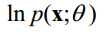。
2，用对数似然函数对参数θ求二阶导数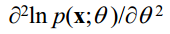3，如果结果依赖于x[n]，则求期望，否则跳过。这个期望就是费雪信息。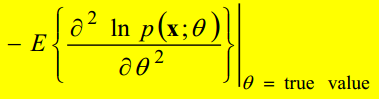连续函数则为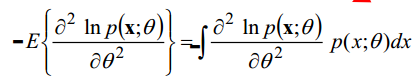注意这里的期望是仅仅是对每个x[n]求取的，同时该期望不是求这N个观测量的平均，而是理论期望。例如，如果x[n]为依赖于n的正太分布N(kn , σ2)，那么x[n]的期望就是kn。如果不知道期望，应该也可以就用x[n]近似，因为其期望也是其若干采样的平均。
4，求费雪信息的倒数即可得到CRLB下界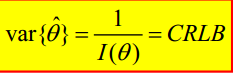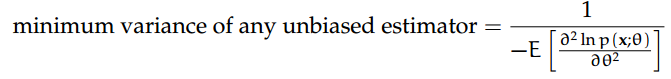实际的CRLB可能跟参数θ本身有关，那么则需要先知道（预估）θ（仿真的时候一般知道实际参数值，实际实验应该只能先估计θ），再计算其预估精度CRLB。
另外，有的函数导数比较复杂，很难求导，这样可以用数值方法求取一阶二阶导数：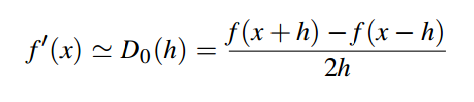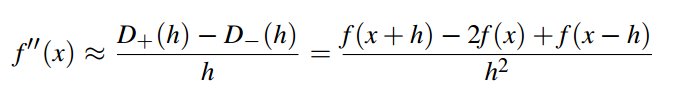举个例子（自创）
求定位成像参数估计得最佳精度该图案为相机拍摄到的单分子成像图案，可以用一个高斯函数近似其强度分布（点扩散函数PSF）。只考虑泊松分布的散粒噪声（shot noise）。
总共有5个参数：A（峰值强度），x0, y0 (高斯函数横向和纵向的位置），σ（PSF宽度），B（背景强度）。
推导过程如下（字写得太丑，请见谅）：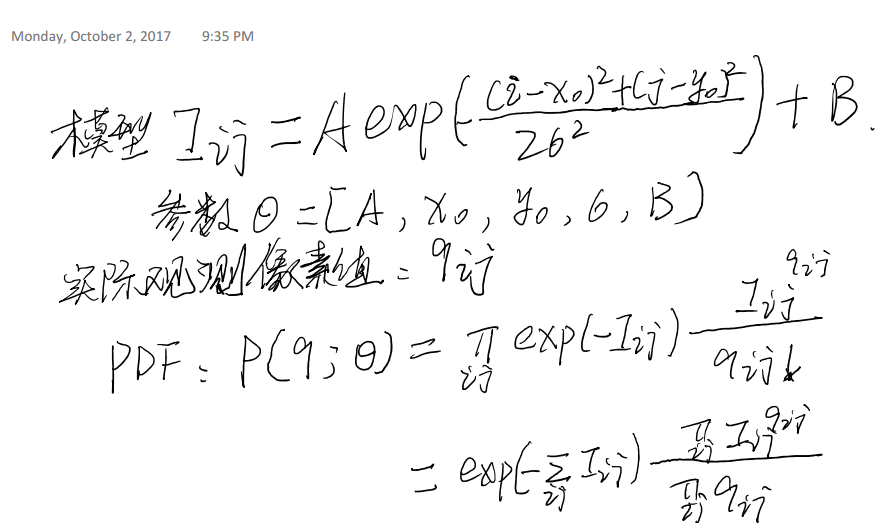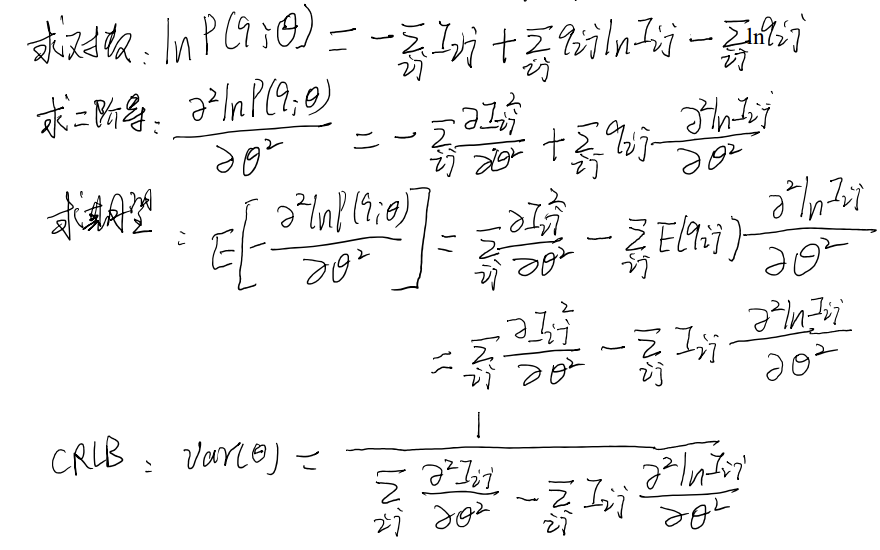这里二阶导基本上只能用数值微分来求解。
后续的优化是用精确的PSF，而不是高斯的PSF，后续则完全一样。当然还可以考虑读出噪声。
展开全文• ## 克拉美罗下界 CRLB的计算

万次阅读 多人点赞 2017-10-03 11:16:34
Cramer-Rao Lower Bound (CRLB)下界可以用于计算无偏估计中能够获得的最佳估计精度，因此经常用于计算理论能达到的最佳估计精度，和评估参数估计方法的性能（是否接近CRLB下界）。这里选择上传了几个讲解非常清楚的...
       克拉美罗下界 Cramer-Rao Lower Bound (CRLB)可以用于计算无偏估计中能够获得的最佳估计精度，因此经常用于计算理论能达到的最佳估计精度，和评估参数估计方法的性能（是否接近CRLB下界）。这里选择上传了几个讲解非常清楚的PPT：http://download.csdn.net/download/u013701860/10006266
总的来说，CRLB的计算为以下几个步骤：
1，构建N的观测量x[n]与估计参数θ的联合概率密度函数 p(X ; θ)=Π p(x[n] ; θ)，然后求对数，得到对数似然函数。
2，用对数似然函数对参数θ求二阶导数3，如果结果依赖于x[n]，则求期望，否则跳过。这个期望就是费雪信息。连续函数则为注意这里的期望是仅仅是对每个x[n]求取的，同时该期望不是求这N个观测量的平均，而是理论期望。例如，如果x[n]为依赖于n的正太分布N(kn , σ2)，那么x[n]的期望就是kn。如果不知道期望，应该也可以就用x[n]近似，因为其期望也是其若干采样的平均。
4，求费雪信息的倒数即可得到CRLB下界实际的CRLB可能跟参数θ本身有关，那么则需要先知道（预估）θ（仿真的时候一般知道实际参数值，实际实验应该只能先估计θ），再计算其预估精度CRLB。
另外，有的函数导数比较复杂，很难求导，这样可以用数值方法求取一阶二阶导数：举个例子（自创）
求定位成像参数估计得最佳精度该图案为相机拍摄到的单分子成像图案，可以用一个高斯函数近似其强度分布（点扩散函数PSF）。只考虑泊松分布的散粒噪声（shot noise）。
总共有5个参数：A（峰值强度），x0, y0 (高斯函数横向和纵向的位置），σ（PSF宽度），B（背景强度）。
推导过程如下（字写得太丑，请见谅）：这里二阶导基本上只能用数值微分来求解。
后续的优化是用精确的PSF，而不是高斯的PSF，后续则完全一样。当然还可以考虑读出噪声。
展开全文精度 极大似然估计
• 三、克拉美罗下界（Cramer_Rao bound）   讨论克拉美罗下界是为了使用这个标准来衡量无偏估计量的性能，克拉美罗下界没有具体的估计方式，只是来反映用已有信息估计参数的最好效果。   总的来说，估计一个参数...
一、正则条件   正则条件是指在陈述某一个定理时，常常需要限定这些定理的使用范围。如果超出这个范围，则会导致定理所描述的内容不成立。用于限定这个使用范围的限定条件被称为“正则条件”。
二、有偏、无偏估计   无偏估计： 估计量的数学期望等于被估参数的真实值，则称此估计量为被估参数的无偏估计。(例：样本均值的期望等于总体均值，所以样本均值为无偏估计）   有偏估计：估计量的数学期望不等于被估参数的真实值，则为有偏估计。(例：样本方差的期望是有偏估计）
举例：   某市所有的高三学生进行了一次数学联考，考试成绩服从1~150的正态分布，那么他们的平均分是多少？   如果将所有学生的成绩累和起来计算均值，这个值叫做总体期望，计算量较为庞大（现实中我们往往不这样计算，只通过采集样本估计）   所以现在给全市的50所高中每一所打一个电话，让他们随机选一名学生的成绩报上来，这样就得到了50个学生的成绩，这50个学生的成绩就是取到的第一个样本组，从而可以算出样本组1的平均数；   再重新给全市50所高中打一个电话，让他们随机选一名学生的成绩报上来，这样又得到了50个学生的成绩，这是取到的第二个样本组，可算出样本组2的平均数；   继续给全市50所高中打一个电话，让他们随机选一名学生的成绩报上来，这样又得到了第三组50个学生的成绩，这是取到第三组样本，同样算出样本组3的平均数；   继续打电话，到最后得到了十组样本的平均数。这时发现这十组样本的平均数符合正态分布，此时就可以用最大似然数估计求得这个正态分布的期望。而十组样本的平均数的期望(均值)，就极其接近总体的期望，这称之为无偏估计（unbiased estimation）。而将单独每一组样本的平均数说是总体平均值的做法，就是有偏估计（biased estimation）。
三、克拉美罗下界（Cramer_Rao bound）   讨论克拉美罗下界是为了使用这个标准来衡量无偏估计量的性能，克拉美罗下界没有具体的估计方式，只是来反映用已有信息估计参数的最好效果。   总的来说，估计一个参数，根据已有信息得到了似然函数，这个似然函数“尖锐”程度（曲率）的倒数（即对数似然函数的二阶导的倒数）就是克拉美罗下界。克拉美罗下界的计算不依赖具体的估计方式，它作为一个用来衡量估计方式好坏的标准，即估计量的方差越靠近克拉美罗下界，效果越好（图像越尖锐、曲率值越大）。   关于克拉美罗下界的更为详细的介绍，参考以下链接：   统计信号处理，简单看看克拉美罗界   克拉美罗下界，CRLB的计算
展开全文有偏估计 无偏估计 正则条件
• 导函数是克拉美罗下界的遗传算法多变量 不会 淘宝太贵 不划算 这里求合理价位matlab
• CRLB是衡量一个无偏估计器是否有效的重要工具，也就是说，给定一个无偏估计器，我们可以利用克拉美-罗下界去判断这个估计器是否是最优的。 3. Example：线性高斯模型（Linear Gaussian model） x = h θ + w , ...
1. 估计量的衡量标准
对于参数估计问题，目前存在着很多估计算法。那么如何去衡量一个估计器（estimator, 也称估计量或估计算法）的性能，我们主要考量以下三个方面
无偏性(unbiased)。对于参数估计问题，设未知参数

θ

\theta

，估计器模型

θ

^

\hat{\theta}

。则有

E

[

θ

^

]

=

θ

\mathbb{E}[\hat{\theta}]=\theta

。对于估计对象为随机变量，则有

E

[

θ

^

]

=

E

[

θ

]

\mathbb{E}[\hat{\theta}]=\mathbb{E}[\theta]

。我们称满足这个条件的估计量为无偏估计量。有效性(availability)。有效性刻画估计量到真实值的偏离程度，

D

(

θ

^

)

=

E

[

(

θ

^

−

E

[

θ

^

]

)

2

]

D(\hat{\theta})=\mathbb{E}[(\hat{\theta}-\mathbb{E}[\hat{\theta}])^2]

，即若存在多种无偏估计器，我们称估计量方差最小的估计器是有效的。一致性(consistency)。设

θ

^

\hat{\theta}

为未知参数

θ

\theta

的估计量，若当样本数

N

→

∞

N\rightarrow \infty

时，对于任意

ϵ

>

0

\epsilon>0

，有

lim

⁡

N

→

∞

P

{

∣

θ

^

−

θ

∣

<

ϵ

}

=

1

\lim\limits_{N\rightarrow \infty} P\left\{{|\hat{\theta}-\theta|<\epsilon}\right\}=1

。我们称

θ

^

\hat{\theta}

与

θ

\theta

是一致的。一致性所体现的是，当样本总数逐渐增加时，估计量逐渐收敛于真实值。
基于这三点考量，那么很自然我们会问，如何衡量一个无偏估计器是否是有效的。统计信号处理理论中的克拉美罗下界（Cramer-Rao Lower Bound，CRLB）就是衡量一个无偏估计器的有力工具。
2. 克拉美-罗下界（Scale Parameter 标量参数）
对于估计参数

θ

\theta

为标量时，假定PDF满足**“正则”**条件

E

[

∂

ln

⁡

p

(

x

;

θ

)

∂

θ

]

=

0

(

for any

θ

)

\mathbb{E}\left[{\frac{\partial \ln p(\boldsymbol{x};\theta)}{\partial \theta}}\right]=0\quad (\ \text{for any }\theta \ )

其中数学期望对

p

(

x

;

θ

)

p(\boldsymbol{x};\theta)

取。那么无偏估计量

θ

^

\hat{\theta}

的方差必然满足

D

(

θ

^

)

≥

1

−

E

[

∂

2

ln

⁡

p

(

x

;

θ

)

∂

θ

2

]

=

1

E

[

(

∂

ln

⁡

p

(

x

;

θ

)

∂

θ

)

2

]

D(\hat{\theta}) \geq \frac{1}{-\mathbb{E}\left[{ \frac{\partial ^2\ln p(\boldsymbol{x};\theta)}{\partial \theta^2}}\right]}=\frac{1}{\mathbb{E}\left[{ \left(\frac{\partial \ln p(\boldsymbol{x};\theta)}{\partial \theta}\right)^2}\right]}

其中导数是在

θ

\theta

的真实值处求，数学期望是对

p

(

x

;

θ

)

p(\boldsymbol{x};\theta)

取。因此，我们可以说一个无偏估计量

g

(

x

)

g(\boldsymbol{x})

达到CRLB，当且仅当，该估计量满足

∂

ln

⁡

p

(

x

;

θ

)

∂

θ

=

I

(

θ

)

(

g

(

x

)

−

θ

)

\frac{\partial \ln p(\boldsymbol{x};\theta)}{\partial \theta} =\mathbf{I}(\theta)(g(\boldsymbol{x})-\theta)

其中，

I

(

θ

)

=

−

E

[

∂

2

ln

⁡

p

(

x

;

θ

)

∂

θ

2

]

\mathbf{I}(\theta)=-\mathbb{E}\left[{ \frac{\partial ^2\ln p(\boldsymbol{x};\theta)}{\partial \theta^2}}\right]

，称为Fisher information。证明见附录A。
Remarks:  CRLB是衡量一个无偏估计器是否有效的重要工具，也就是说，给定一个无偏估计器，我们可以利用克拉美-罗下界去判断这个估计器是否是最优的。
3. Example：线性高斯模型（Linear Gaussian model）

x

=

h

θ

+

w

,

w

∼

N

(

0

,

C

w

)

其中

θ

\theta

是未知参数，

x

∈

R

p

\boldsymbol{x}\in \mathbb{R}^p

是观测值（observed signal），

w

\boldsymbol{w}

是均值为

0

\boldsymbol{0}

，协方差矩阵为

C

w

\boldsymbol{C}_{\boldsymbol{w}}

的高斯噪声。
我们考虑如下估计器

θ

^

=

(

h

T

C

w

−

1

h

)

−

1

h

T

C

w

−

1

x

\hat{\theta}=(\boldsymbol{h}^T\boldsymbol{C}_{\boldsymbol{w}}^{-1}\boldsymbol{h})^{-1}\boldsymbol{h}^T\boldsymbol{C}_{\boldsymbol{w}}^{-1}\boldsymbol{x}

对于该模型，其似然函数

p

(

x

;

θ

)

p(\boldsymbol{x};\theta)

为

p

(

x

;

θ

)

=

1

(

2

π

)

p

/

2

∣

C

w

∣

1

/

2

exp

⁡

[

−

1

2

(

x

−

h

θ

)

T

C

w

−

1

(

x

−

h

θ

)

]

p(\boldsymbol{x};\theta)=\frac{1}{(2\pi)^{p/2}|\boldsymbol{C}_{\boldsymbol{w}}|^{1/2}} \exp \left[{-\frac{1}{2}(\boldsymbol{x}-\boldsymbol{h}\theta)^T\boldsymbol{C}_{\boldsymbol{w}}^{-1}(\boldsymbol{x}-\boldsymbol{h}\theta)}\right]

因此
无偏性

E

[

θ

^

]

=

∫

x

θ

^

p

(

x

;

θ

)

d

x

\mathbb{E}[\hat{\theta}]=\int_{\boldsymbol{x}} \hat{\theta} p(\boldsymbol{x};\theta)\text{d}\boldsymbol{x}

我们可以将

p

(

x

;

θ

)

p(\boldsymbol{x};\theta)

看作为自变量为

x

\boldsymbol{x}

均值为

h

θ

\boldsymbol{h}\theta

，协方差矩阵为

C

w

\boldsymbol{C}_{\boldsymbol{w}}

的高斯PDF，即

∫

x

x

p

(

x

;

θ

)

d

x

=

h

θ

\int_{\boldsymbol{x}}\boldsymbol{x}p(\boldsymbol{x};\theta)\text{d}\boldsymbol{x}=\boldsymbol{h}\theta

。因此

E

[

θ

^

]

=

(

h

T

C

w

−

1

h

)

−

1

h

T

C

w

−

1

h

θ

=

θ

\mathbb{E}[\hat{\theta}]=(\boldsymbol{h}^T\boldsymbol{C}_{\boldsymbol{w}}^{-1}\boldsymbol{h})^{-1}\boldsymbol{h}^T\boldsymbol{C}_{\boldsymbol{w}}^{-1}\boldsymbol{h}\theta=\theta

，即

θ

^

\hat{\theta}

为无偏估计量。  有效性

∂

ln

⁡

p

(

x

;

θ

)

∂

θ

=

(

x

−

h

θ

)

T

C

w

−

1

h

\frac{\partial \ln p(\boldsymbol{x};\theta)}{\partial \theta}=(\boldsymbol{x}-\boldsymbol{h}\theta)^T\boldsymbol{C}_{\boldsymbol{w}}^{-1}\boldsymbol{h}

∂

2

ln

⁡

p

(

x

;

θ

)

∂

θ

2

=

−

h

T

C

w

−

1

h

\frac{\partial^2 \ln p(\boldsymbol{x};\theta)}{\partial \theta^2}=-\boldsymbol{h}^T\boldsymbol{C}_{\boldsymbol{w}}^{-1}\boldsymbol{h}

关于矩阵求导不太熟悉的朋友可以看下这个网站：https://en.wikipedia.org/wiki/Matrix_calculus。 基于上述表述，该系统模型的CRLB为

−

1

−

E

[

∂

2

ln

⁡

p

(

x

;

θ

)

∂

θ

2

]

=

1

h

T

C

w

−

1

h

-\frac{1}{-\mathbb{E}\left[{ \frac{\partial ^2\ln p(\boldsymbol{x};\theta)}{\partial \theta^2}}\right]}=\frac{1}{\boldsymbol{h}^T\boldsymbol{C}_{\boldsymbol{w}}^{-1}\boldsymbol{h}}

而估计器

θ

^

\hat{\theta}

的方差为

D

(

θ

^

)

=

(

(

h

T

C

w

−

1

h

)

−

1

h

T

C

w

−

1

)

C

w

(

(

h

T

C

w

−

1

h

)

−

1

h

T

C

w

−

1

)

T

=

(

h

T

C

w

−

1

h

)

−

1

D(\hat{\theta})=\left({(\boldsymbol{h}^T\boldsymbol{C}_{\boldsymbol{w}}^{-1}\boldsymbol{h})^{-1}\boldsymbol{h}^T\boldsymbol{C}_{\boldsymbol{w}}^{-1}}\right) \boldsymbol{C}_{\boldsymbol{w}} \left({(\boldsymbol{h}^T\boldsymbol{C}_{\boldsymbol{w}}^{-1}\boldsymbol{h})^{-1}\boldsymbol{h}^T\boldsymbol{C}_{\boldsymbol{w}}^{-1}}\right)^T=(\boldsymbol{h}^T\boldsymbol{C}_{\boldsymbol{w}}^{-1}\boldsymbol{h})^{-1}

由于

h

T

C

w

−

1

h

\boldsymbol{h}^T\boldsymbol{C}_{\boldsymbol{w}}^{-1}\boldsymbol{h}

是一维的，有

(

h

T

C

w

−

1

h

)

−

1

=

1

h

T

C

w

−

1

h

(\boldsymbol{h}^T\boldsymbol{C}_{\boldsymbol{w}}^{-1}\boldsymbol{h})^{-1}=\frac{1}{\boldsymbol{h}^T\boldsymbol{C}_{\boldsymbol{w}}^{-1}\boldsymbol{h}}

，因此，该估计量是有效的，即该无偏估计量

θ

\theta

的方差可以达到CRLB。  一致性 将系统模型

x

=

h

θ

+

w

\boldsymbol{x}=\boldsymbol{h}\theta+\boldsymbol{w}

代入估计器中，有

θ

^

=

(

h

T

C

w

−

1

h

)

−

1

h

T

C

w

−

1

(

h

θ

+

w

)

=

θ

+

(

h

T

C

w

−

1

h

)

−

1

h

T

C

w

−

1

w

\hat{\theta} =(\boldsymbol{h}^T\boldsymbol{C}_{\boldsymbol{w}}^{-1}\boldsymbol{h})^{-1}\boldsymbol{h}^T\boldsymbol{C}_{\boldsymbol{w}}^{-1}(\boldsymbol{h}\theta+\boldsymbol{w})\\ =\theta+(\boldsymbol{h}^T\boldsymbol{C}_{\boldsymbol{w}}^{-1}\boldsymbol{h})^{-1}\boldsymbol{h}^T\boldsymbol{C}_{\boldsymbol{w}}^{-1}\boldsymbol{w}

若假设噪声能量一定，即

C

w

\boldsymbol{C}_{\boldsymbol{w}}

元素值固定，随着观测样本

p

→

∞

p\rightarrow\infty

，则噪声的方差

D

(

(

h

T

C

w

−

1

h

)

−

1

h

T

C

w

−

1

w

)

=

1

h

T

c

w

−

1

h

D((\boldsymbol{h}^T\boldsymbol{C}_{\boldsymbol{w}}^{-1}\boldsymbol{h})^{-1}\boldsymbol{h}^T\boldsymbol{C}_{\boldsymbol{w}}^{-1}\boldsymbol{w})=\frac{1}{\boldsymbol{h}^T\boldsymbol{c}_{\boldsymbol{w}}^{-1}\boldsymbol{h}}

从公式可以看出，假设噪声

w

\boldsymbol{w}

的每个元素具有相同的方差，则必然

lim

⁡

p

→

∞

h

T

c

w

−

1

h

→

∞

\lim\limits_{p\rightarrow \infty}\boldsymbol{h}^T\boldsymbol{c}_{\boldsymbol{w}}^{-1}\boldsymbol{h}\rightarrow \infty

。因此，当

p

→

∞

p\rightarrow \infty

时，我们可以将估计量

θ

^

\hat{\theta}

看作

θ

^

=

θ

+

n

,

n

∼

N

(

0

,

(

h

T

C

w

−

1

h

)

−

1

)

and

lim

⁡

p

→

∞

h

T

c

w

−

1

h

→

∞

\hat{\theta}=\theta+n,\quad n\sim\mathcal{N}(0,(\boldsymbol{h}^T\boldsymbol{C}_\boldsymbol{w}^{-1}\boldsymbol{h})^{-1}) \ \ \text{and} \ \lim\limits_{p\rightarrow \infty}\boldsymbol{h}^T\boldsymbol{c}_{\boldsymbol{w}}^{-1}\boldsymbol{h}\rightarrow \infty

因此，对于任意

ϵ

>

0

\epsilon>0

，有

lim

⁡

N

→

∞

P

{

∣

θ

^

−

θ

∣

<

ϵ

}

=

1

\lim\limits_{N\rightarrow \infty} P\left\{{|\hat{\theta}-\theta|<\epsilon}\right\}=1

即，该估计量满足一致性。
4. CRLB证明
由于

θ

^

\hat{\theta}

是无偏估计，即

∫

x

(

θ

^

−

θ

)

p

(

x

;

θ

)

d

x

=

0

⇒

∫

θ

^

p

(

x

;

θ

)

d

x

=

θ

注意，估计器

θ

^

\hat{\theta}

是关于观测量

x

\boldsymbol{x}

的函数。上式等式两边对

θ

\theta

求偏导有

∫

θ

^

∂

p

(

x

;

θ

)

∂

θ

d

x

=

1

⇒

∫

θ

^

∂

ln

⁡

p

(

x

;

θ

)

∂

θ

p

(

x

;

θ

)

d

x

=

1

−

−

−

(

∗

1

)

由正则条件

E

[

∂

ln

⁡

p

(

x

;

θ

)

∂

θ

]

=

0

\mathbb{E}\left[\frac{\partial \ln p(\boldsymbol{x};\theta)}{\partial \theta}\right]=0

，即

∫

∂

ln

⁡

p

(

x

;

θ

)

∂

θ

p

(

x

;

θ

)

d

x

=

0

\int \frac{\partial \ln p(\boldsymbol{x};\theta)}{\partial \theta} p(\boldsymbol{x};\theta)\text{d}\boldsymbol{x}=0

有

θ

∫

∂

ln

⁡

p

(

x

;

θ

)

∂

θ

p

(

x

;

θ

)

d

x

=

0

⇒

∫

θ

∂

ln

⁡

p

(

x

;

θ

)

∂

θ

p

(

x

;

θ

)

d

x

=

0

−

−

−

(

∗

2

)

\theta \int \frac{\partial \ln p(\boldsymbol{x};\theta)}{\partial \theta} p(\boldsymbol{x};\theta)\text{d}\boldsymbol{x}=0\\ \qquad \qquad \Rightarrow \int \theta \frac{\partial \ln p(\boldsymbol{x};\theta)}{\partial \theta} p(\boldsymbol{x};\theta)\text{d}\boldsymbol{x}=0---(*2)

合并（*1）与（*2）,有

∫

(

θ

^

−

θ

)

∂

ln

⁡

p

(

x

;

θ

)

∂

θ

p

(

x

;

θ

)

d

x

=

1

⇒

∫

(

θ

^

−

θ

)

p

(

x

;

θ

)

∂

ln

⁡

p

(

x

;

θ

)

∂

θ

p

(

x

;

θ

)

d

x

=

1

\ \ \ \ \int (\hat{\theta}-\theta)\frac{\partial \ln p(\boldsymbol{x};\theta)}{\partial \theta}p(\boldsymbol{x};\theta)\text{d}\boldsymbol{x}=1\\ \qquad \qquad \ \ \Rightarrow \int (\hat{\theta}-\theta)\sqrt{p(\boldsymbol{x};\theta)}\frac{\partial \ln p(\boldsymbol{x};\theta)}{\partial \theta}\sqrt{p(\boldsymbol{x};\theta)}\text{d}\boldsymbol{x}=1

由于柯西-施瓦茨不等式

∫

f

2

(

x

)

d

x

∫

g

2

(

x

)

d

x

≥

(

∫

f

(

x

)

g

(

x

)

d

x

)

2

\int f^2(x)\text{d}x \int g^2(x)\text{d}x \geq\left({\int f(x)g(x)\text{d}x}\right)^2

当且仅当

f

(

x

)

=

g

(

x

)

f(x)=g(x)

时，取等号。
根据柯西-施瓦茨不等式（Cauchy-Schwarz inequality），有

(

∫

(

θ

^

−

θ

)

2

p

(

x

;

θ

)

d

x

)

(

∫

(

∂

ln

⁡

p

(

x

;

θ

)

∂

θ

)

2

p

(

x

;

θ

)

d

x

)

≥

1

⇒

∫

(

θ

^

−

θ

)

2

p

(

x

;

θ

)

d

x

≥

1

(

∫

(

∂

ln

⁡

p

(

x

;

θ

)

∂

θ

)

2

p

(

x

;

θ

)

d

x

)

\left({ \int (\hat{\theta}-\theta)^2{p(\boldsymbol{x};\theta)}\text{d}\boldsymbol{x}}\right) \left({\int \left(\frac{\partial \ln p(\boldsymbol{x};\theta)}{\partial \theta}\right)^2p(\boldsymbol{x};\theta)\text{d}\boldsymbol{x}}\right)\geq 1\\ \Rightarrow \int (\hat{\theta}-\theta)^2{p(\boldsymbol{x};\theta)}\text{d}\boldsymbol{x}\geq \frac{1}{\left({\int \left(\frac{\partial \ln p(\boldsymbol{x};\theta)}{\partial \theta}\right)^2p(\boldsymbol{x};\theta)\text{d}\boldsymbol{x}}\right)}

即

D

(

θ

^

)

≥

1

E

[

(

∂

ln

⁡

p

(

x

;

θ

)

∂

θ

)

2

]

D(\hat{\theta})\geq \frac{1}{\mathbb{E}\left[{\left(\frac{\partial \ln p(\boldsymbol{x};\theta)}{\partial \theta}\right)^2}\right]}

现在只需证明

E

[

(

∂

ln

⁡

p

(

x

;

θ

)

∂

θ

)

2

]

=

−

E

[

∂

2

ln

⁡

p

(

x

;

θ

)

∂

θ

2

]

\mathbb{E}\left[{\left(\frac{\partial \ln p(\boldsymbol{x};\theta)}{\partial \theta}\right)^2}\right]=-\mathbb{E}\left[{ \frac{\partial ^2\ln p(\boldsymbol{x};\theta)}{\partial \theta^2}}\right]

证：由正则条件

E

[

∂

ln

⁡

p

(

x

;

θ

)

∂

θ

]

=

0

\mathbb{E}\left[{\frac{\partial \ln p(\boldsymbol{x};\theta)}{\partial \theta}}\right]=0

，等式两边对

θ

\theta

求偏导，有

∂

∂

θ

∫

∂

ln

⁡

p

(

x

;

θ

)

∂

θ

p

(

x

;

θ

)

d

x

=

0

⇒

∫

[

∂

2

ln

⁡

p

(

x

;

θ

)

∂

θ

2

p

(

x

;

θ

)

+

∂

ln

⁡

p

(

x

;

θ

)

∂

θ

∂

p

(

x

;

θ

)

∂

θ

]

d

x

=

0

⇒

∫

∂

2

ln

⁡

p

(

x

;

θ

)

∂

θ

2

p

(

x

;

θ

)

d

x

=

−

∫

(

∂

ln

⁡

p

(

x

;

θ

)

∂

θ

)

2

p

(

x

;

θ

)

d

x

\frac{\partial }{\partial \theta} \int \frac{\partial \ln p(\boldsymbol{x};\theta)}{\partial \theta} p(\boldsymbol{x};\theta)\text{d}\boldsymbol{x}=0\quad \qquad \qquad \qquad \ \\ \quad \Rightarrow \int \left[{\frac{\partial^2 \ln p(\boldsymbol{x};\theta)}{\partial \theta^2}p(\boldsymbol{x};\theta)+\frac{\partial \ln p(\boldsymbol{x};\theta)}{\partial \theta}\frac{\partial p(\boldsymbol{x};\theta)}{\partial \theta}}\right]\text{d}\boldsymbol{x}=0\\ \qquad \ \Rightarrow \int \frac{\partial^2 \ln p(\boldsymbol{x};\theta)}{\partial \theta^2}p(\boldsymbol{x};\theta)\text{d}\boldsymbol{x}=-\int \left({\frac{\partial \ln p(\boldsymbol{x};\theta)}{\partial \theta}}\right)^2p(\boldsymbol{x};\theta)\text{d}\boldsymbol{x}

现在证明，若估计量

θ

^

=

g

(

x

)

\hat{\theta}=\text{g}(\boldsymbol{x})

可以达到CRLB，则有

∂

ln

⁡

p

(

x

;

θ

)

∂

θ

=

I

(

θ

)

(

g

(

x

)

−

θ

)

\frac{\partial \ln p(\boldsymbol{x};\theta)}{\partial \theta} =\mathbf{I}(\theta)(g(\boldsymbol{x})-\theta)

其中，

I

(

θ

)

=

−

E

[

∂

2

ln

⁡

p

(

x

;

θ

)

∂

θ

2

]

\mathbf{I}(\theta)=-\mathbb{E}\left[{ \frac{\partial ^2\ln p(\boldsymbol{x};\theta)}{\partial \theta^2}}\right]

。
证：等式两边同时对

θ

\theta

求偏导，有

∂

2

ln

⁡

p

(

x

;

θ

)

∂

θ

2

=

∂

I

(

θ

)

∂

θ

(

g

(

x

)

−

θ

)

−

I

(

θ

)

\frac{\partial^2 \ln p(\boldsymbol{x};\theta)}{\partial \theta^2} =\frac{\partial \mathbf{I}(\theta)}{\partial \theta}(g(\boldsymbol{x})-\theta)-\mathbf{I}(\theta)

等式两边同时对乘上

p

(

x

;

θ

)

p(\boldsymbol{x};\theta)

，并对

x

\boldsymbol{x}

积分，得

E

[

∂

2

ln

⁡

p

(

x

;

θ

)

∂

θ

2

]

=

−

I

(

θ

)

\mathbb{E}\left[{\frac{\partial^2 \ln p(\boldsymbol{x};\theta)}{\partial \theta^2} }\right]=-\mathbf{I}(\theta)

证毕。
展开全文• 然后用利用CRLB判断剩下的无偏估计量中哪个最接近下界，选取最接近下界的估计量作为最优的无偏估计量。 注意： 一个估计量是MVUE，但不一定是有效的估计量；反之，有效的估计量一定是MVUE 。如下图： 图片来源...
• MALTLAB仿真克拉美罗界，CRLB即不可能求得方差小于下限的无偏估计量，并为比较无偏估计量的性能提供了一个标准。
• 本文档通俗易懂讲解的讲解了Cramer-Rao下界（克拉美罗下界），适合初学者
• 克拉美-罗下界（Cramer-Rao Lower Bound，CRLB）： 衡量一个无偏估计器好坏的有力工具。
• 针对宽带LFM信号的DOA估计问题，从宽带LFM信号模型的描述人手提出且详细分析了在该模型下基于分数阶Fourier变换的DOA估计算法。并以计算机仿真实验结果为依据，表明该算法与时频—相干信号子空间算法相比，...罗下界
• 统计信号估计中，克拉美罗下界实际上是对无偏估计量给出了方差的下界，也就是说，只要你使用无偏估计，方差一定大于等于CRLB统计学
• 克拉美罗下界 Cramer-Rao Lower Bound (CRLB)可以用于计算无偏估计中能够获得的最佳估计精度，因此经常用于计算理论能达到的最佳估计精度，和评估参数估计方法的性能（是否接近CRLB下界）。 本次上传内容为：MIMO...
• 这个下界就是克拉美-（Cramer-Rao）界。 1.5.2.1 非随机参量估计的边界 设 是非随机参量  的任意无偏估计，则有 当且仅当对所有 和 都满足 时等号成立。其中 可以是 的函数，但不能是 和 的...
• 概率论基础一、概率密度函数1.1 定义1.2 贝叶斯公式及推断1.3 矩1.4 样本均值和样本方差1.5 统计独立性与不相关性1.6 归一化积1.7 负熵和互信息1.8 克拉美罗下界和费歇尔信息量二、高斯概率密度函数2.1 定义2.2 ...
• 在对通信系统估计问题的最新科研文章的调研中，我发现CRLB （克拉美罗下界）的出镜率非常高。这也说明了这一早就被提出的概念，至今能追随着潮流，也值得去掌握。 因此，简单地写了这篇文章来概述下 CRLB 与 科研中...
• 首先,建立双线阵模型,其中使用了克拉美罗下界分析其性能极限。然后,分析了延时相加波束形成(即平面阵波束形成)和延时相减波束形成,并讨论了模型失配的影响和处理,随后给出实际声纳系统中的处理流程。最后,给出采用...
• 针对信道退化的多跳无线传感器网络... 利用信道退化统计量和译码策略, 推导出二元观测数据的似然函数, 基于最大似然估计获得目标定位, 进一步给出目标位置估计的克拉美-罗下界. 仿真结果表明了所提出算法的有效性.</p>
• 然后以随机分布粒子计算新生目标检测概率,以后验克拉美-罗下界(PCRLB)预测长时跟踪精度,以隐马尔可夫模型(HMM)滤波器推导长时辐射代价;最后构建新生目标检测概率和已有目标跟踪精度约束下辐射控制的长时优化函数,给...
• 我们对克拉美-罗下界进行了后期计算，结果表明，对于足够数量的视图，估计参数的样本方差从经验上可达到克拉美-罗下界。此外，我们将校准结果与独立的地面真实性进行了比较，并观察到，随着视野数量的增加，平均误差...
• https://www.cnblogs.com/rubbninja/p/4512765.html 转载于:https://www.cnblogs.com/focus-z/p/10402446.html
• HBF系统的导频接收表示 对于一个窄带的混合波束成形系统， 导频接收信号可表示为： Y=WHVST+WZ(1)\mathbf{Y}=\mathbf{W} \mathbf{H} \mathbf{V} \mathbf{S}^{T}+\mathbf{W Z} \tag{1}Y=WHVST+WZ(1) ...
• 又称Cramer-Rao lower bounds(CRLB)，克拉美-罗下界。 克拉美罗界是对于参数估计问题提出的，为任何无偏估计量的方差确定了一个下限。无偏估计量的方差只能无限制的逼近CRB，而不会低于CRB，因此这个界也可以称为...
• 接（2），给出CRLB推导 在实际的定位过程中，常用的TDOA定位方法的克拉美-罗下界为 其中，Q 为 TDOA 测量值误差矢量的协方差矩阵，c 表示电磁信号在空气中的传播速度。G 是与目标...
• Cramer-Rao Lower Bound (CRLB)下界可以用于计算无偏估计中能够获得的最佳估计精度，因此经常用于计算理论能达到的最佳估计精度，和评估参数估计方法的性能（是否接近CRLB下界）。本篇博客融合和总结了若干PPT的内容...